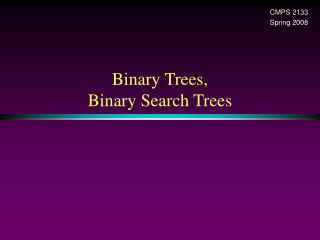DownloadDownload PresentationBinary Trees, Binary Search Trees

# Binary Trees, Binary Search Trees

Download Presentation## Binary Trees, Binary Search Trees

- - - - - - - - - - - - - - - - - - - - - - - - - - - E N D - - - - - - - - - - - - - - - - - - - - - - - - - - -
##### Presentation Transcript

1. CMPS 2133 Spring 2008 Binary Trees,Binary Search Trees

2. Trees • Linear access time of linked lists is prohibitive • Does there exist any simple data structure for which the running time of most operations (search, insert, delete) is O(log N)?

3. Trees • A tree is a collection of nodes • The collection can be empty • (recursive definition) If not empty, a tree consists of a distinguished node r (the root), and zero or more nonempty subtrees T1, T2, ...., Tk, each of whose roots are connected by a directed edge from r

4. Some Terminologies • Child and parent • Every node except the root has one parent  • A node can have an arbitrary number of children • Leaves • Nodes with no children • Sibling • nodes with same parent

5. Some Terminologies • Path • Length • number of edges on the path • Depthof a node • length of the unique path from the root to that node • The depth of a tree is equal to the depth of the deepest leaf • Height of a node • length of the longest path from that node to a leaf • all leaves are at height 0 • The height of a tree is equal to the height of the root • Ancestor and descendant • Proper ancestor and proper descendant

6. Example: UNIX Directory

7. Binary Trees • A tree in which no node can have more than two children • The depth of an “average” binary tree is considerably smaller than N, eventhough in the worst case, the depth can be as large as N – 1.

8. Example: Expression Trees • Leaves are operands (constants or variables) • The other nodes (internal nodes) contain operators • Will not be a binary tree if some operators are not binary

9. Tree traversal • Used to print out the data in a tree in a certain order • Pre-order traversal • Print the data at the root • Recursively print out all data in the left subtree • Recursively print out all data in the right subtree

10. Preorder, Postorder and Inorder • Preorder traversal • node, left, right • prefix expression • ++a*bc*+*defg

11. Preorder, Postorder and Inorder • Postorder traversal • left, right, node • postfix expression • abc*+de*f+g*+ • Inorder traversal • left, node, right. • infix expression • a+b*c+d*e+f*g

12. Preorder

13. Postorder

14. Preorder, Postorder and Inorder

15. Binary Trees • Possible operations on the Binary Tree ADT • parent • left_child, right_child • sibling • root, etc • Implementation • Because a binary tree has at most two children, we can keep direct pointers to them

16. compare: Implementation of a general tree

17. Binary Search Trees • Stores keys in the nodes in a way so that searching, insertion and deletion can be done efficiently. • Binary search tree property • For every node X, all the keys in its left subtree are smaller than the key value in X, and all the keys in its right subtree are larger than the key value in X

18. Binary Search Trees A binary search tree Not a binary search tree

19. Binary search trees Two binary search trees representing the same set: • Average depth of a node is O(log N); maximum depth of a node is O(N)

20. Implementation

21. Searching BST • If we are searching for 15, then we are done. • If we are searching for a key < 15, then we should search in the left subtree. • If we are searching for a key > 15, then we should search in the right subtree.

22. Searching (Find) • Find X: return a pointer to the node that has key X, or NULL if there is no such node • Time complexity • O(height of the tree)

23. Inorder traversal of BST • Print out all the keys in sorted order Inorder: 2, 3, 4, 6, 7, 9, 13, 15, 17, 18, 20

24. findMin/ findMax • Return the node containing the smallest element in the tree • Start at the root and go left as long as there is a left child. The stopping point is the smallest element • Similarly for findMax • Time complexity = O(height of the tree)

25. insert • Proceed down the tree as you would with a find • If X is found, do nothing (or update something) • Otherwise, insert X at the last spot on the path traversed • Time complexity = O(height of the tree)

26. delete • When we delete a node, we need to consider how we take care of the children of the deleted node. • This has to be done such that the property of the search tree is maintained.

27. delete • Three cases: • (1) the node is a leaf • Delete it immediately • (2) the node has one child • Adjust a pointer from the parent to bypass that node

28. delete • (3) the node has 2 children • replace the key of that node with the minimum element at the right subtree • delete the minimum element • Has either no child or only right child because if it has a left child, that left child would be smaller and would have been chosen. So invoke case 1 or 2. • Time complexity = O(height of the tree)